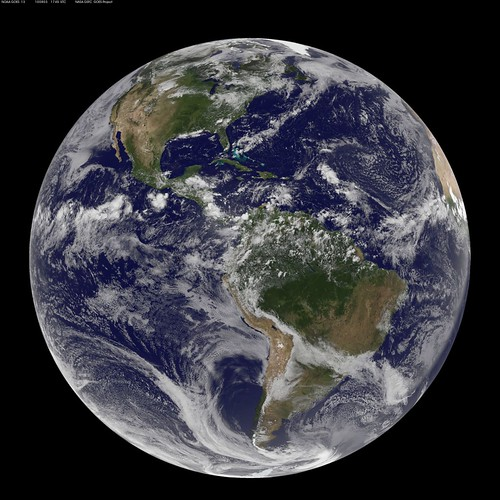# Weight and centrifugal force

Confusingly, weight is defined differently in physics than in the rest of the world! To a physicist, weight is the force that an object of a certain mass exerts as a result of gravity, defined by this equation:

W = mg (W is weight in Newtons, m is mass in kg, and g is the acceleration due to gravity).

What we actually mean by ‘weight’ is a physicist’s ‘mass’: when we weigh ourselves on scales, the value that it shows is our mass. So although you might be weightless out in space, you still have a mass that is the same wherever you go.

So what has this to do with centripetal force? Centripetal force is the inwards force on an object which is rotating around an axis. Think of it in terms of a ball on the end of a string: imagine twirling the ball around your head. The string is taut and holds the ball so that is spins in a circle, rather than just flying off. Centripetal force is that tension in the string, holding the ball on its circular path. It’s proportional to the velocity (speed, but with direction) of the rotating object squared, and inversely proportional to the radius of the orbit (distance from the centre). This means that if the velocity increases, the force increases (a lot), and if the radius increases, the force decreases.

However, although centripetal force acts inwards on the ball, if you were sitting on the ball you’d feel like you were being pulled outside the circle rather than towards it. It’s the same on any theme park ride, or roundabout: you feel like you’re being pushed out of the circle. This is due to centrifugal force, an apparent force that results from seeing things from the perspective of the rotating object, and is proportional to the distance from the centre of the circle – completely the opposite of the centripetal force.Image: NOAA/NASA GOES Project

So, back to weight! As the Earth is rotating, all of us are in fact ‘balls on a string’: held in a circular orbit around the Earth’s centre by the pull of gravity, the centripetal force. But, as we’re rotating, we experience a centrifugal force, so there seems to be a force acting against gravity!

Now imagine a bird’s eye view of the Earth, spinning around the axis, which goes through the poles. On the pole, the distance from you to the centre will be zero, and will increase until you reach the equator. So, the distance from you to the centre changes depending on where you are on the Earth, which means so does the centrifugal force you experience.

Since the centrifugal force acts against gravity, this means that you will actually experience slightly less downwards force on the equator than on the poles. And since weight depends on the downwards force you experience, you will weigh less, and fall less fast, on the equator than on the poles!

Advertisements